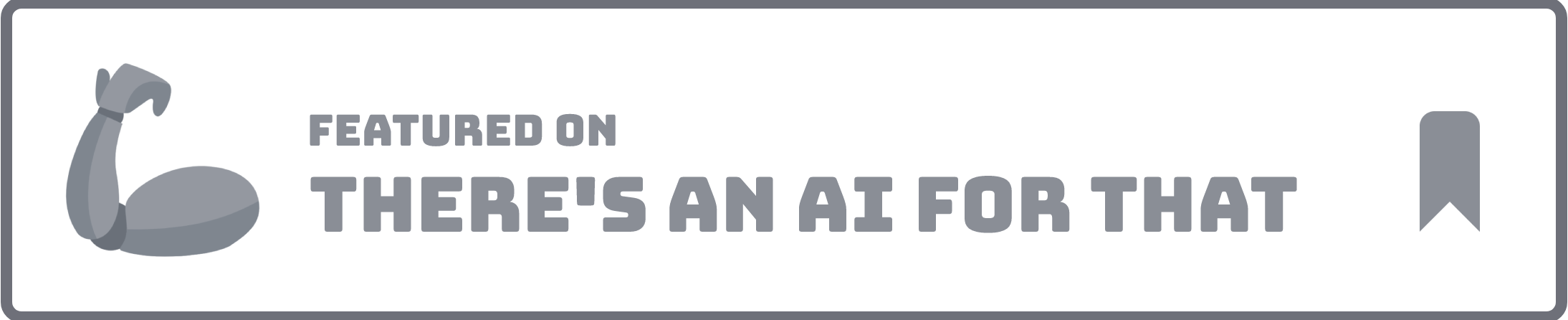Excel formulas 2022-12-04# Formula Dog

1.0(1)
Excel formulas generated with assistance.
Overview
Generated by ChatGPT

Formula Dog is an AI-powered tool designed to help users quickly and easily generate Excel formulas, VBA code, and regex from their text instructions. The tool is designed to be intuitive and user-friendly, automatically interpreting user instructions and providing real-time feedback.

Formula Dog also provides users with the ability to convert code, formulas, and SQL into English, making it easier to understand complex formulas. Additionally, Formula Dog is developing a Microsoft Excel add-on and has a Google Sheets add-on that is currently under review.

The tool is designed to save users time and energy in creating and understanding formulas, and can be tested for free.

Formula Dog was manually vetted by our editorial team and was first featured on December 9th 2022.Promote this AI Claim this AI

## Would you recommend Formula Dog?

Help other people by letting them know if this AI was useful.

## 20 alternatives to Formula Dog for Excel formulas

•Generated and explained Excel/Sheets formulas.
1,318
1
5.0
•Creating complex Excel formulas and macros.
113
•82
•56
1
•47
•Instant Excel formula generation from text instructions.
45
•Simplified complex calculations in Excel.
44
1
5.0
•Simplified creation and understanding of Excel formulas.
35
•Analyzed data, collaborated, improved productivity.
31
•Excel formula suite for smarter productivity.
29
•25
•Streamlined Excel formula generation from instructions.
25
3
3.0
•Automates Excel formula creation with explanations.
21
•Summarizes and categorizes text in spreadsheets.
14
•Improved productivity through automating Excel/Sheets.
14
•Formula & Query Gen. for Spreadsheets & Databases.
10
•Excel formulas
8
•Excel productivity with efficient formula generation.
8
•Excel Bot simplifies formulas & generates VBA code
7
•Data analysis, formulas, and preparation streamlined.
6

## Pros and Cons

### Pros

Generates Excel formulas
Creates VBA code
Generates regex
Interprets user instructions
Provides real time feedback
Converts code to English
Converts formulas to English
Converts SQL to English
Saves time and energy
Free to test
Turns text into formulas
Turns text into VBA code
Generates regex from English
Explains formulas, code, SQL
From English to MS Excel
From English to Airtable
From English to VBA Code
Quick access from MS Excel
Helps understand complex formulas

### Cons

No Linux support
No mobile version
Microsoft Excel add-on still in development
No offline functionality
No API noted
No multi-language support
Cannot handle complex queries
Limited format support
Free trial only, unknown price

## Q&A

What is Formula Dog?
How does Formula Dog generate Excel formulas?
Can Formula Dog interpret VBA code from my text instructions?
What is the role of AI in Formula Dog?
What feedback does Formula Dog provide in real-time?
Does Formula Dog convert code, formulas, and SQL into English?
Is there a Microsoft Excel add-on being developed by Formula Dog?
What is the status of the Google Sheets add-on for Formula Dog?
Can Formula Dog assist in understanding complex Excel formulas?
Does Formula Dog support Regex generation from English instructions?
Can Formula Dog explain what a SQL code means?
Is there an Airtable integration with Formula Dog?
How does Formula Dog assist in turning my thoughts into Excel formulas?
How can Formula Dog turn my thoughts into VBA code?
Can Formula Dog provide explanations for the generated VBA code?
What kind of user interface does Formula Dog provide?
Does Formula Dog support English to Regex conversions?
How does Formula Dog save time in creating Excel formulas?
What are the future developments planned for Formula Dog?

## If you liked Formula Dog

•1,318
1
5.0
•51
5.0
•4
•3
•2
•23
••3
•70
•12
•55
1
2.0
•36
3
5.0

## People also searched

### Help

+ D bookmark this site for future reference
+ ↑/↓ go to top/bottom
+ ←/→ sort chronologically/alphabetically
Enter open selected entry in new tab
⇧ + Enter open selected entry in new tab
⇧ + ↑/↓ expand/collapse list
/ focus search
Esc remove focus from search
A-Z go to letter (when A-Z sorting is enabled)
+ submit an entry